equilibrium level of national income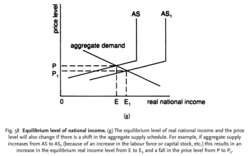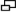Fig. 58 Equilibrium level of national income. (g) The equilibrium level of real national income and the price level will also change if there is a shift in the aggregate supply schedule. For example, if aggregate supply increases from AS to AS1 (because of an increase in the labour force or capital stock, etc.) this results in an increase in the equilibrium real income level from E to E1 and a fall in the price level from P to P1 .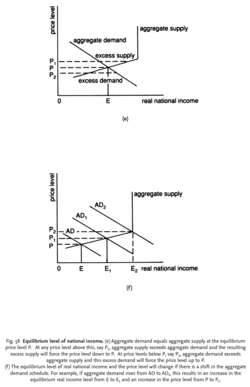Fig. 58 Equilibrium level of national income. (e) Aggregate demand equals aggregate supply at the equilibrium price level P. At any price level above this, say P1, aggregate supply exceeds aggregate demand and the resulting excess supply will force the price level down to P. At price levels below P, say P2, aggregate demand exceeds aggregate supply and this excess demand will force the price level up to P.

(f) The equilibrium level of real national income and the price level will change if there is a shift in the aggregate demand schedule. For example, if aggregate demand rises from AD to AD1, this results in an increase in the equilibrium real income level from E to E1 and an increase in the price level from P to P 1 .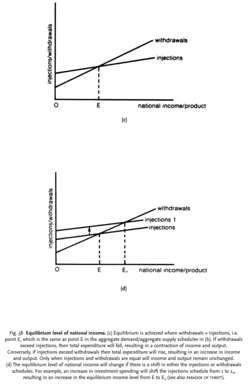Fig. 58 Equilibrium level of national income. (c) Equilibrium is achieved where withdrawals = injections, i.e. point E, which is the same as point E in the aggregate demand/aggregate supply schedules in (b). If withdrawals exceed injections, then total expenditure will fall, resulting in a contraction of income and output. Conversely, if injections exceed withdrawals then total expenditure will rise, resulting in an increase in income and output. Only when injections and withdrawals are equal will income and output remain unchanged.

(d) The equilibrium level of national income will change if there is a shift in either the injections or withdrawals schedules. For example, an increase in investment spending will shift the injections schedule from 1 to 1 1, resulting in an increase in the equilibrium income level from E to E1 (See also PARADOX OF THRIFT).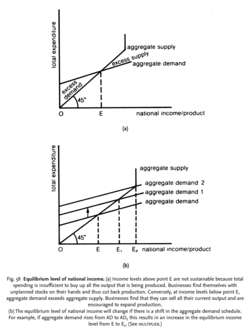Fig. 58 Equilibrium level of national income. (a) Income levels above point E are not sustainable because total spending is insufficient to buy up all the output that is being produced. Businesses find themselves with unplanned stocks on their hands and thus cut back production. Conversely, at income levels below point E, aggregate demand exceeds aggregate supply. Businesses find that they can sell all their current output and are encouraged to expand production.

(b) The equilibrium level of national income will change if there is a shift in the aggregate demand schedule. For example, if aggregate demand rises from AD to AD1 this results in an increase in the equilibrium income level from E to E1. (See MULTIPLIER.)

equilibrium level of national income

1. the level of NATIONAL INCOME at which the purchasing and production plans of the economy are synchronized. This occurs at the point of intersection of the AGGREGATE DEMAND SCHEDULE with the AGGREGATE SUPPLY SCHEDULE, which is point E in Fig. 58 Equilibrium income is not necessarily the level of income at which FULL EMPLOYMENT is attained, for an equilibrium level of income can occur at any level of economic activity. Full employment equilibrium is a special case where aggregate demand exactly corresponds with POTENTIAL GROSS NATIONAL PRODUCT, leaving no INFLATIONARY GAP or DEFLATIONARY GAP. For example, aggregate demand 2 in Fig. 58 (b) represents a full employment equilibrium where E2 corresponds with full employment output.
2. the level of national income at which total INJECTIONS (investment + government expenditure + exports) is exactly equal to WITHDRAWALS (saving + taxes + imports). Also called injections-withdrawals approach to national income determination. In the CIRCULAR FLOW OF NATIONAL INCOME MODEL, income = consumption + withdrawals, spending = consumption + injections. See Fig. 58 (c) and (d).
3. the level of real national income at which aggregate demand is exactly equal to aggregate supply as shown by the intersection of the aggregate demand curve and the aggregate supply curve at a particular PRICE LEVEL. See Fig. 58 (e), (f) and (g).
References in periodicals archive ?
The neoclassical direction of causation was from the labor market, which determined the equilibrium level of employment, to the real goods market, where, in conjunction with aggregate supply, the equilibrium level of national income was determined [Johnson and Cate, 2002; Johnson et al.
The composition of national income determines the level of aggregate demand and the equilibrium level of national income in the real goods market.
The second unique feature of Keynes' equilibrium was that it reflected his belief that the composition of national income determined the level of aggregate demand, which in turn, was the primary determinant of the equilibrium level of national income and employment in the short-run.
The neoclassical direction of causation was from the labor market, which determined the equilibrium level of employment to the real goods market, where, in conjunction with aggregate supply, the equilibrium level of national income was determined.

Site: Follow: Share:
Open / Close